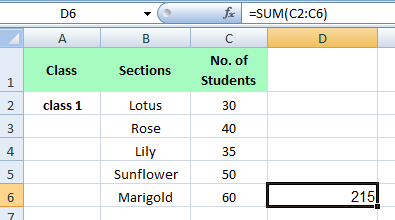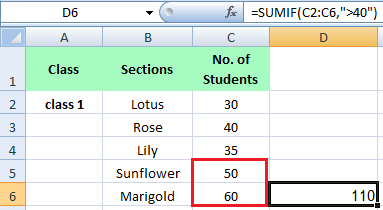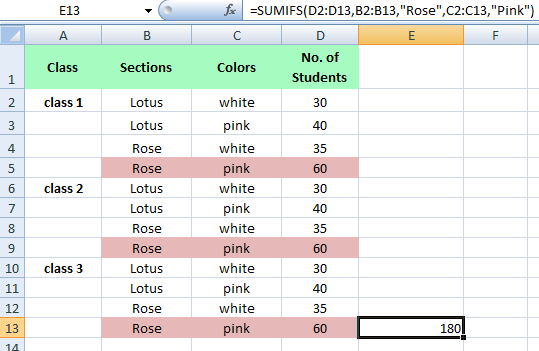### Sum Function in MS Excel

The Sum function is one of the most used functions in MS Excel. This function let you sum the cells based on one or multiple criteria.

### How to use SUM Function in MS Excel

The "Sum" function is useful in many types of Worksheets like Receipts, Budgets and any kind of worksheet that requires cumulative total for all values within range.
In the SUM Function we will learn how to use

• ### SUMIFS

How to use SUM function
Let's learn with Example

SUM function is used to sum the range of cells.
D6 equals =SUM(C2:C6)How to use SUMIF function
Let's learn with Example

SUMIF function is used to sum cells based on one criteria .
For example, Sum the values greater than 40
D6 equals =SUMIF(C2:C6,”>40”)How to use SUMIFS function
Let's learn with Example
SUMIFS function is used to sum cells based on multiple criteria.
For example, Sum the values of Rose and Pink
E13 equals =SUMIFS(D2:D13,B2:B13,"Rose",C2:C13,"Pink")